## Quiz 10 : Profit MaximizationLooking for Economics Homework Help?# Quiz 10 : Profit Maximization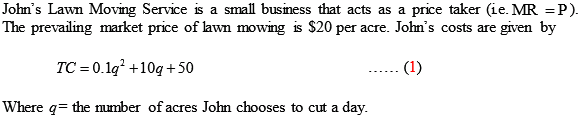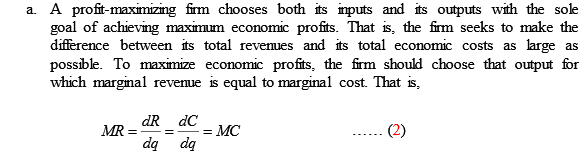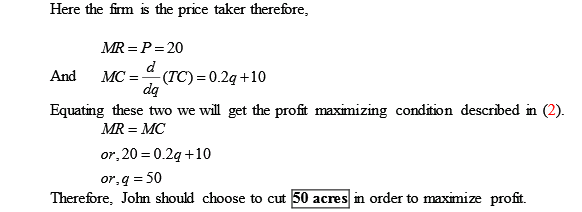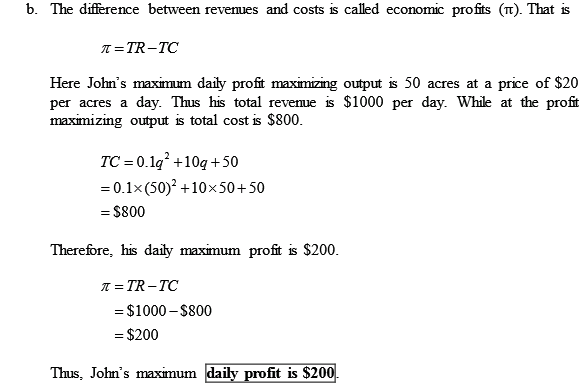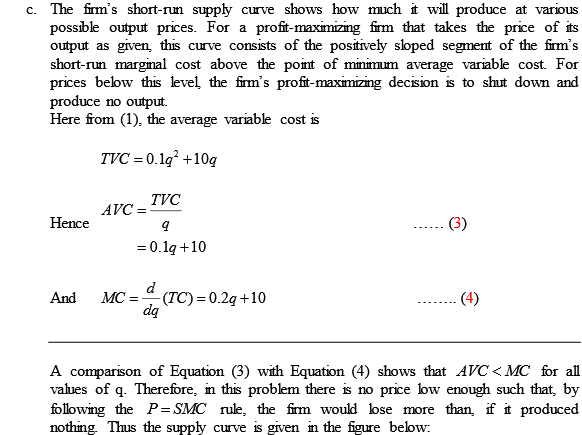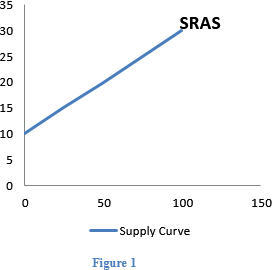It is given that the cost function of the widget production is TC =0.25 q 2 where q = q A + q L. The two nations that demand widgets are nation- A and nation- L. The demand of widgets in nation- A is given as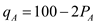and the demand of widgets in nation- L is given as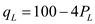. Nation- A : The profit maximizing point will be attained at a point where MR=MC. The price is calculated from demand function as shown below: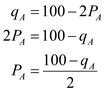The total revenue for widget in America is given below: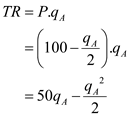The marginal revenue is the derivative of TR. The derivation is shown below: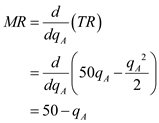The marginal cost is derivative of total cost with respect to q as shown below: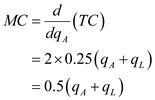Equate the marginal revenue to marginal cost. The calculation is shown below: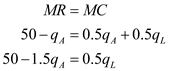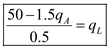…… (1) Nation- L Similarly, for maximizing profit in nation- L the firm should choose that output for which marginal revenue is equal to marginal cost. The price is calculated from demand function as shown below: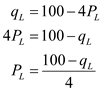The total revenue is given below: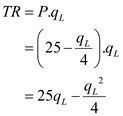The marginal revenue is derivative of TR as shown below: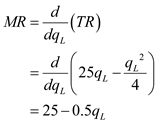The marginal cost is derivative of total cost with respect to q. That is,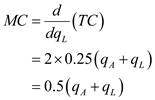Evaluate the marginal revenue to marginal cost as shown below: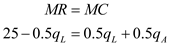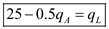…… (2) Equate equation (1) and (2).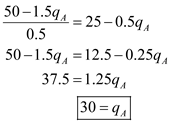Thus, the profit maximizing level of output in nation- A is 30 units. Substitute this value of output in nation- A 's inverse demand function.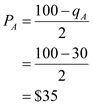Thus, the profit maximizing price in nation- A is \$35 per unit. Substitute the value of output in equation (1) to get the optimal production level in nation- L.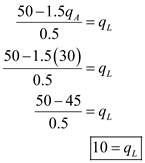Thus, the profit maximizing level of output in nation- L is 10 units. Substitute this value of output in nation- L 's inverse demand function.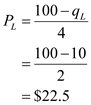Thus, the profit maximizing price in nation- L is \$22.5 per unit. Conclusion: The firm must sell 30 units in nation- A and 10 units in nation- L to maximize profits. The price charged by the firm is \$35 per unit in nation- A and \$22.5 per unit in nation- L.

The production function for a calculator producing firm is given as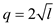Here,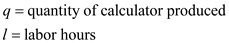It is also given that: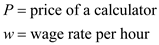(a) In order to calculate the total cost function for a calculator producing firm, first the value of l in terms of q is computed. The value of l is computed as shown below: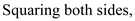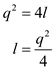Thus, value of l is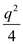. ……. (1) The total cost function represents the cost incurred by the firm in production of calculators. It is wage rate times the labor hours used to produce calculator. The value of l is taken from (1). The total cost function is calculated as shown below: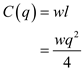Therefore, the total cost function is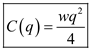. (b) The profit function shows the difference between the total revenue earned by selling calculators and the cost incurred to produce them. The calculation of profit function is shown as follows: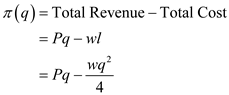Thus, the profit function for the firm given is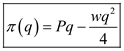. (c) The level of output at which the firm maximizes its profit, gives the supply function for the firm. At given wage level and price, the supply function for the given firm is derived as shown below: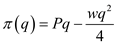Differentiating with respect to q ,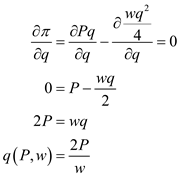…… (2) Therefore, the supply function is given as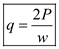. (d) The demand function of labor for the firm can be calculated as follows: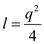Take the value of q from equation (2)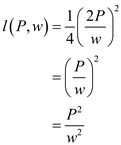Thus, demand curve of labor for the firm is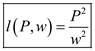. (e) The function obtained shows a positive relation between labor demanded and the price of calculator. The same relation holds for true for supply of calculators by the firm and price charged. This is so because if the price increases for the calculator, producer will be willing to increase its supply too. And hence, demand more labor, which is a factor of production. Both functions have inverse relationship with the wage rate. This is because with increase in wages, producer reduces their demand for labor and supply for calculator also decreases.

There is no answer for this question